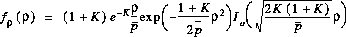### JPL's Wireless Communication Reference Website# Distribution of Signal Strength for Rician Fading

Expressed in terms of the local-mean power and the Rician K-factor, the pdf of the signal amplitude becomesThis Special Purpose Embedded Calculator calculates the distribution, i.e., the pdf integrated from zero to a thrshold value. This presents the probability that a signal amplitude drops below that threshold value.

### Input parameters:

K factor
Power in LOS divided by
scattered power.
dB
LOS+scattered power divided by
minimum required power (threshold).
dB

### Result:

Outage Probability
• Note 1: enter a large negative number (e.g. -100 dB) for the K factor to model Rayleigh fading.
• Note 2: Do you see a probability larger than unity? For small outage values, there is an exponent ("e-..") in the outage probability. Some browsers may hide this outside the visible field if they present too many mantissa bits. If you are in doubt, place the cursor in the Outage Probability field and move to the right, beyond the visibile area. Any exponent will scroll into the window.
• Convert LOS amplitudes and sigma to the K-factor and power.